# High School Math : How to find the equation of a curve

## Example Questions

### Example Question #1 : How To Find The Equation Of A Curve

What line goes through points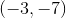and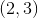?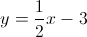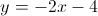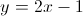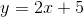Explanation:

First, we find the slope between the two points: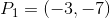and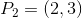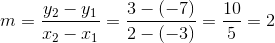Plug the slope and one point into the slope-intercept form to calculate the intercept: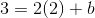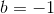Thus the equation between the points becomes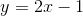.